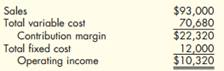# Contribution Margin Ratio, Variable Cost Ratio, Break-Even Sales Revenue The controller of Andreston Company prepared the following projected income statement: Required: 1. Calculate the contribution margin ratio. 2. Calculate the variable cost ratio. 3. Calculate the break-even sales revenue for Andreston. 4. Conceptual Connection: How could Andreston increase projected operating income without increasing the total sales revenue?

Question

Contribution Margin Ratio, Variable Cost Ratio, Break-Even Sales Revenue The controller of Andreston Company prepared the following projected income statement:Required:

1. Calculate the contribution margin ratio.

2. Calculate the variable cost ratio.

3. Calculate the break-even sales revenue for Andreston.

4. Conceptual Connection: How could Andreston increase projected operating income without increasing the total sales revenue?

### Want to see this answer and more?

Experts are waiting 24/7 to provide step-by-step solutions in as fast as 30 minutes!*

*Response times may vary by subject and question complexity. Median response time is 34 minutes for paid subscribers and may be longer for promotional offers.
Tagged in